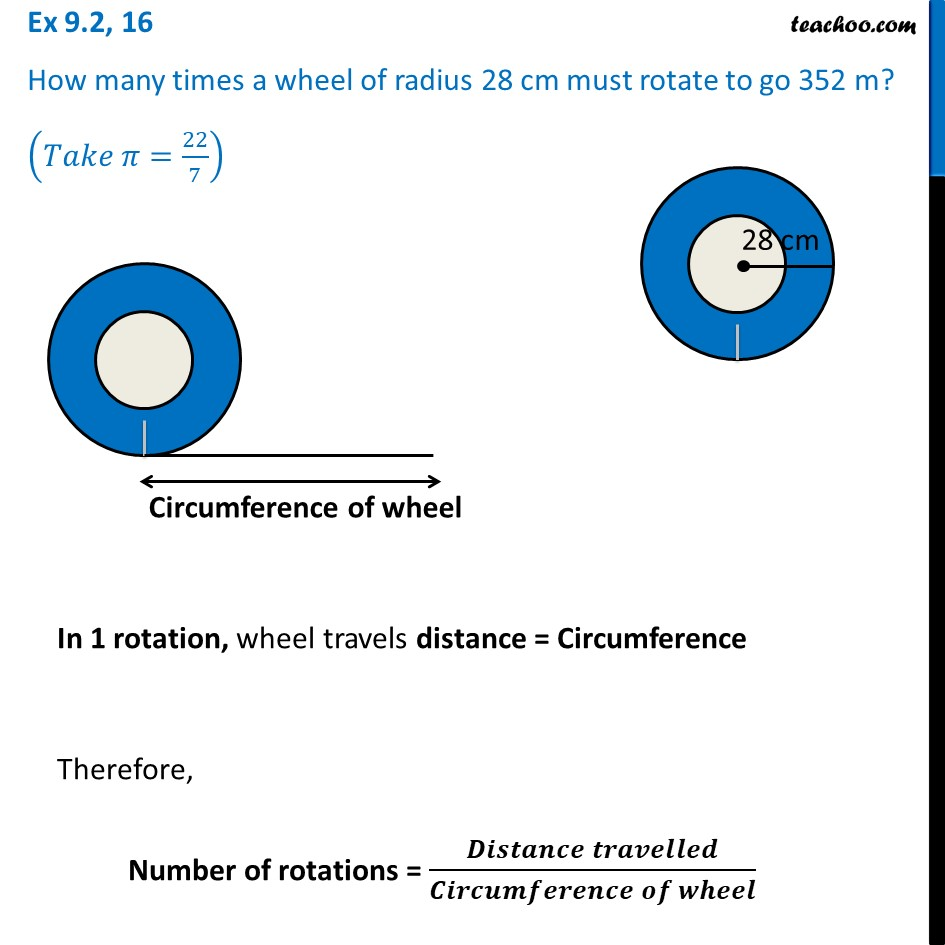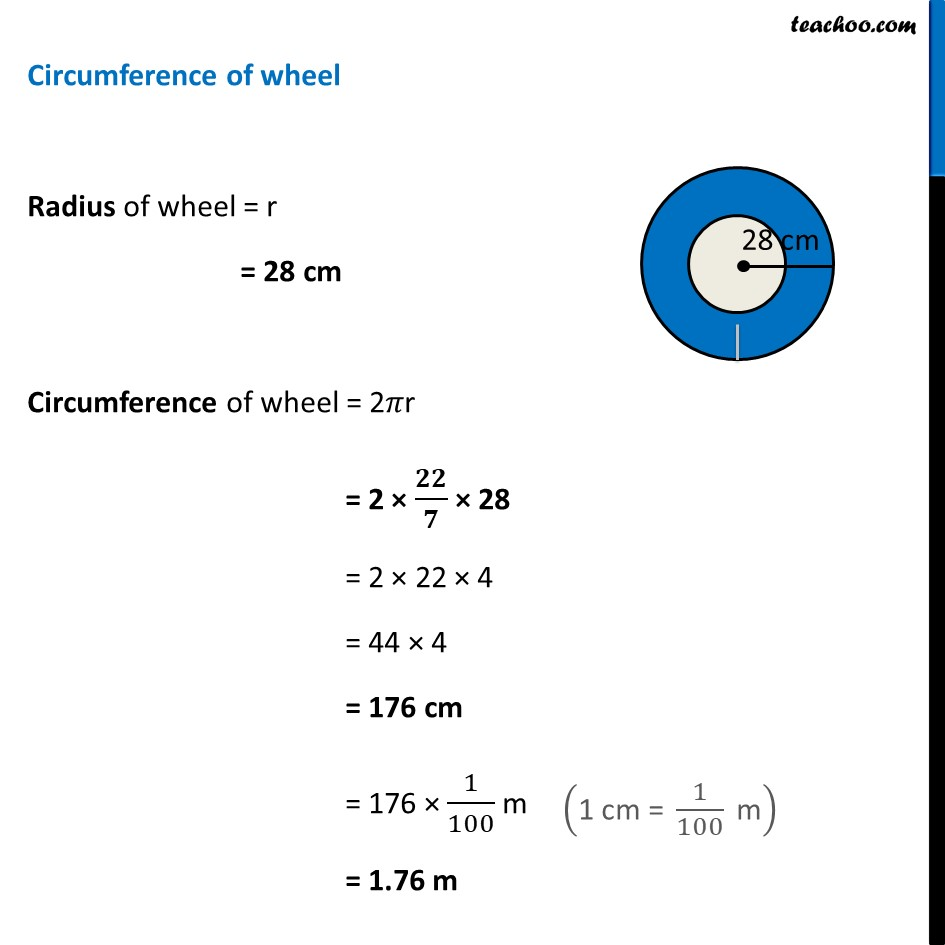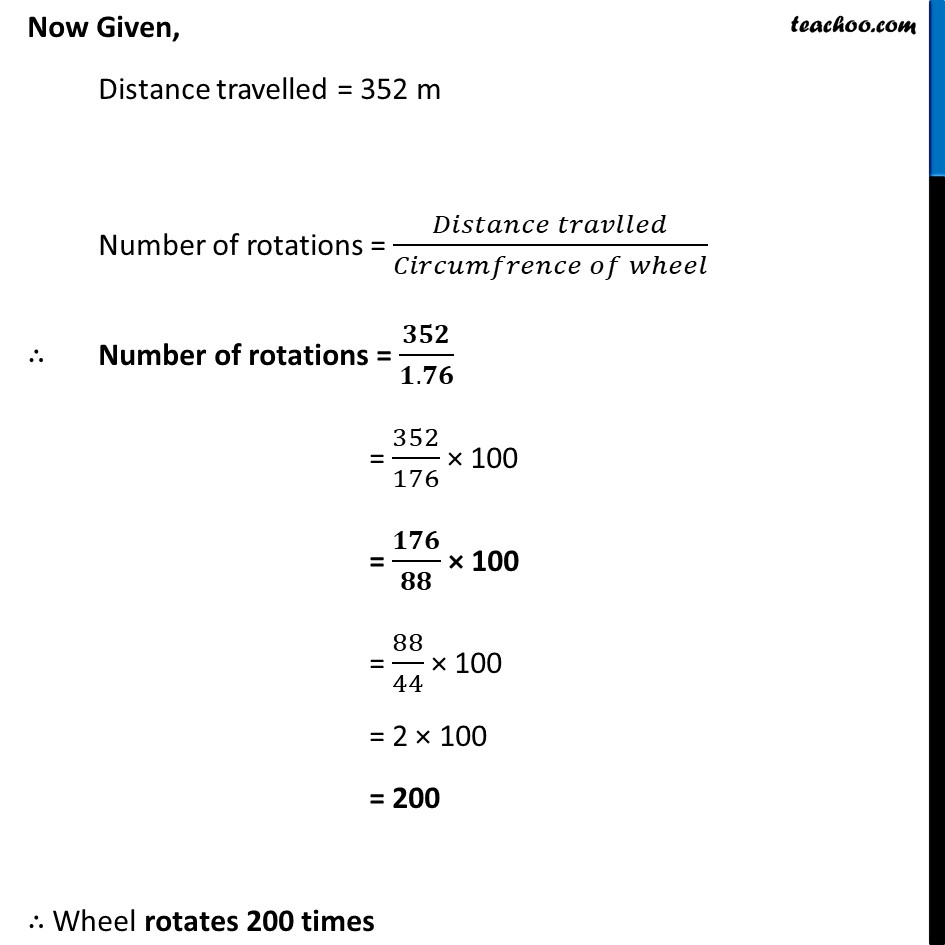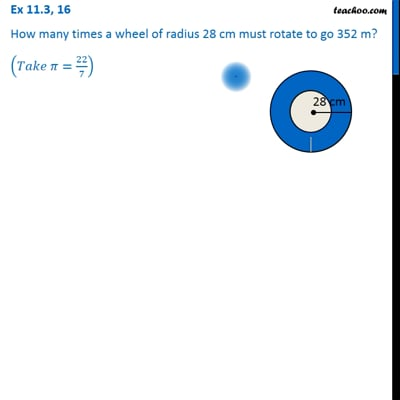Ex 9.2

Chapter 9 Class 7 Perimeter and Area
Serial order wiseThis video is only available for Teachoo black users

Learn in your speed, with individual attention - Teachoo Maths 1-on-1 Class

### Transcript

Ex 9.2, 16 How many times a wheel of radius 28 cm must rotate to go 352 m? (𝑇𝑎𝑘𝑒 𝜋=22/7) In 1 rotation, wheel travels distance = Circumference Therefore, Number of rotations = (𝑫𝒊𝒔𝒕𝒂𝒏𝒄𝒆 𝒕𝒓𝒂𝒗𝒆𝒍𝒍𝒆𝒅)/(𝑪𝒊𝒓𝒄𝒖𝒎𝒇𝒆𝒓𝒆𝒏𝒄𝒆 𝒐𝒇 𝒘𝒉𝒆𝒆𝒍) Circumference of wheel Radius of wheel = r = 28 cm Circumference of wheel = 2𝜋r = 2 × 𝟐𝟐/𝟕 × 28 = 2 × 22 × 4 = 44 × 4 = 176 cm = 176 × 1/100 m = 1.76 m Now Given, Distance travelled = 352 m Number of rotations = (𝐷𝑖𝑠𝑡𝑎𝑛𝑐𝑒 𝑡𝑟𝑎𝑣𝑙𝑙𝑒𝑑)/(𝐶𝑖𝑟𝑐𝑢𝑚𝑓𝑟𝑒𝑛𝑐𝑒 𝑜𝑓 𝑤ℎ𝑒𝑒𝑙) ∴ Number of rotations = 𝟑𝟓𝟐/(𝟏.𝟕𝟔) = 352/176 × 100 = 𝟏𝟕𝟔/𝟖𝟖 × 100 = 88/44 × 100 = 2 × 100 = 200 ∴ Wheel rotates 200 times